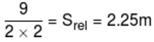Courses

# JEE Main Physics Mock Test

## 25 Questions MCQ Test Mock Test Series for JEE Main & Advanced 2022 | JEE Main Physics Mock Test

Description
This mock test of JEE Main Physics Mock Test for JEE helps you for every JEE entrance exam. This contains 25 Multiple Choice Questions for JEE JEE Main Physics Mock Test (mcq) to study with solutions a complete question bank. The solved questions answers in this JEE Main Physics Mock Test quiz give you a good mix of easy questions and tough questions. JEE students definitely take this JEE Main Physics Mock Test exercise for a better result in the exam. You can find other JEE Main Physics Mock Test extra questions, long questions & short questions for JEE on EduRev as well by searching above.
QUESTION: 1

Solution:
QUESTION: 2

Solution:
QUESTION: 3

### In a transformer, the number of turns in primary coil and secondary coil are 5 and 4 respectively. If 240 V is applied on the primary coil, then the ratio of current in primary and secondary coil is

Solution:

We know that

Ns / Np =  Is / Ip , where p represents primary and s ,secondary coil

Ip / Is = 4 : 5

QUESTION: 4
The wavelength 'λ' for a particle with momentum p
Solution:
QUESTION: 5
A straight wire of length l and electric dipole moment p is bent to form a semicircle. The new dipole moment would be
Solution:
QUESTION: 6
Two charged conducting spheres of radii a and b are connected to each other by a wire. The ratio of electric fields at the surface of two spheres is
Solution:
QUESTION: 7
The net charge on a current carrying conductor is
Solution:
QUESTION: 8
Two pieces of a glass plate, placed one upon the other with a little water in between them, can not be separated easily, because of
Solution:
QUESTION: 9
A ball of mass 0.25 kg attached to the end of a string of length 1.96 m is moving in a horizontal circle. The string will break if the tension is more than 25 N. What is the maximum speed with which the ball can be moved
Solution:
QUESTION: 10

A bullet of mass m and velocity V is fired into a block of mass M and sticks to it. The final velocity would be

Solution:
QUESTION: 11
A logic gate is an electronic circuit which
Solution:
QUESTION: 12

If a person with a spring balance and a body hanging from it goes up and up in an aeroplane, then reading of weight of body as indicated by spring balance will

Solution:

Spring balance measures the weight of the body which depends upon the value of g. The height obtained by an aeroplane will not bring significant change in g so the spring balance is to read the same

QUESTION: 13
In the following question, a Statement of Assertion (A) is given followed by a corresponding Reason (R) just below it. Read the Statements carefully and mark the correct answer-
Assertion(A):A wire has a resistance of 12Ω. It is bent in the form of a circle. The effective resistance between two points on any diameter is 6Ω.
Reason(R): Equivalent resistance between two points on any diameter is in series.
Solution:
QUESTION: 14
In radioactive decay process, the negatively charged emitted $\beta$ -particles are
Solution:
QUESTION: 15
In the following question, a Statement of Assertion (A) is given followed by a corresponding Reason (R) just below it. Read the Statements carefully and mark the correct answer-
Assertion(A): Refractive index of a material depends on the frequency of the light
Reason(R): Incoming e.m. wave applies an electric field and polarises it, contributing to (∈) permittivity of the medium, depending on its frequency.
Solution:
QUESTION: 16
Blowing air with open mouth is an example of
Solution:
QUESTION: 17
A spherical ball rolls on a table without slipping. Then the fraction of its total energy associated with rotation is
Solution:
QUESTION: 18
A stationary point source of sound emits sound uniformly in all directions in a non-absorbing medium. Two points P and Q are at a distance of 4m and 9m respectively from the source. The ratio of amplitudes of the waves at P and Q is
Solution:
QUESTION: 19
Which of the following is a dimensionless quantity?
Solution:
QUESTION: 20

We have two radioactive nuclei A and B. Both convert into a stable nucleus C. Nucleus A converts into Cafter emitting two αα-particles and three β-particles. Nucleus B converts into C after emitting one αα-particle and five β-particles. At time t=0, nuclei of A are 4N0 and that of B are N0. Half life of A (into the conversion of C) is 1min and that of B is 2min. Initially number of nuclei of C are zero.

Q. What are number of nuclei of C, when number of nuclei of A and B are equal?

Solution:
*Answer can only contain numeric values
QUESTION: 21

'n' moles of an ideal gas undergoes a process A → B as shown in the figure. The maximum temperature of the gas during the process will be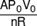Then the value of A is :-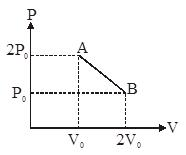Solution:
*Answer can only contain numeric values
QUESTION: 22

In Y.D.S.E. using light of wavelength 6000Å angular fringe width of fringe formed on the screen is 1°. Find the distance between the slits :-

Solution: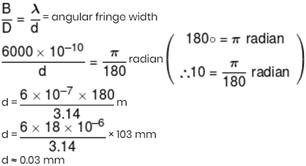*Answer can only contain numeric values
QUESTION: 23

An electron moves in the field produced by a charged sphere of radius 10 cm along the radius connecting the points separated by 12 and 15 cm from the centre of the sphere. The velocity of the electron changes thereby from 2 × 105 to 2 × 106 m/s. Determine the surface charge (in nC/m2) density of the sphere. (Mass of electron = 9.1 × 10–31 kg)

Solution: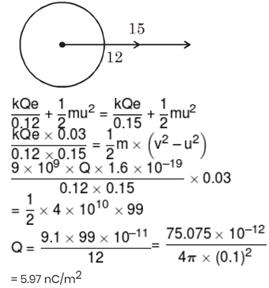*Answer can only contain numeric values
QUESTION: 24

You are given a converging lens with equal radii of curvature and a diverging lens with the same radii of curvature as those of the converging lens. The lenses are made of material with n = 1.50, and the radii of curvature are all 35 cm. They are placed at opposite ends of a tube 15 cm long, and the nearer lens which is converging is 10 cm from an object. What is the location of the image that results from the two refractions? Write the final distance (in cm) from diverging lens.

Solution: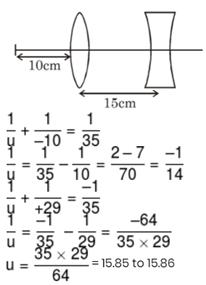*Answer can only contain numeric values
QUESTION: 25

The new white belt of a long horizontal conveyor is moving with a constant speed v = 3.0 m/s. A small block of carbon is placed on the belt with zero initial velocity relative to the ground. The block will slip a bit before moving with the belt, leaving a black mark on the belt (figure). How long is that mark (in m) if the coefficient of kinetic friction between the carbon block and the belt is 0.20 and the coefficient of static friction is 0.30?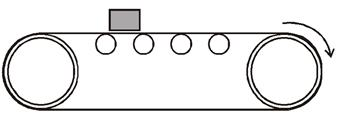Solution:

02 = v2 – 2µkgSrel